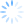CDXZipStream and CDXGeoData provide options for calculating either the straight-line ("as the crow flies") distance or driving (road) distance between locations. When selecting the distance function that best meets the need of an application, calculation time is also a factor; driving distance calculations are much more complex and take significantly longer, and may not be practical for very large data sets. Is straight-line distance a reasonable alternative? We know that straight-line distance always underestimates the actual length of a route (with the exception of routes along a perfectly straight road), but by how much?

To find out we performed an analysis using the functions in CDXGeoData. These functions are CDXGeoDistance for calculating straight-line distance, and CDXGeoRoute, which calculates travel distance and time using Bing Maps as the routing data source. We developed data sets where travel was within large regions in the contiguous U.S., as well as within specific counties. For each county, the start point for each route was at a single ZIP Code and the end point covered every other ZIP Code in the county. Here are the results:Average straight-line underestimation is surprisingly consistent within regional and county areas, ranging about 11 to 18% and 18 to 23% less than the shortest route calculation, respectively.

Underestimation of route distance is greater when compared to the quickest route calculation, along with more standard deviation as well. Straight-line distance versus quickest route is a bit of an apple-to-oranges comparison, but is included here since quickest is usually the preferred travel route. For U.S. regions, straight-line distance versus quickest route is about 15 to 25% less, and excluding Los Angeles County (a probable outlier), county area straight-line distance compared to quickest route ranges about 26 to 30% less.

So for ball-park calculations, such as estimation of shipping costs, it would be realistic to assume straight-line distance plus up to about 30% additional mileage, depending on the route coverage area.  Straight-line distance is more accurate for longer routes probably because both local road and geographic restrictions – such rivers, lakes, parks, and other obstacles – are less important over longer distances. CDXGeodata uses a free Microsoft Excel template which completely automates straight-line distance calculation between ZIP Codes. Here is a short tutorial showing how it works:

For straight-line distance calculations using specific address locations, we offer an Excel template that works with CDXZipStream to generate reports of locations within a specific radius area.  Please watch the video below for a short tutorial:

When working with large data sets that require highly accurate routing analysis, we recommend a two-step process using straight-line distance calculations to first narrow down the list of candidate locations within a radius, then calculating the actual route distances from this smaller list.  Please refer to our post CDXZipStream Straight-line and Driving Distance Calculations for further discussion.